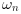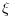Scilab Home page | Wiki | Bug tracker | Forge | Mailing list archives | ATOMS | File exchange
Scilab 6.0.0
Change language to: English - Français - 日本語 - Русский

See the recommended documentation of this function

# damp

Natural frequencies and damping factors.

### Syntax

[wn,z] = damp(sys)
[wn,z] = damp(P [,dt])
[wn,z] = damp(R [,dt])

### Parameters

sys

A linear dynamical system (see syslin).

P

An array of polynomials.

R

An array of real or complex floating point numbers.

dt

A non negative scalar, with default value 0.

wn

vector of floating point numbers in increasing order: the natural pulsation in rad/s.

z

vector of floating point numbers: the damping factors.

### Description

The denominator second order continuous time transfer function with complex poles can be written as s^2 + 2*z*wn*s + wn^2 where z is the damping factor and wn the natural pulsation.

If sys is a continuous time system, [wn,z] = damp(sys) returns in wn the natural pulsation(in rad/s) and in z the damping factorsof the poles of the linear dynamical system sys. The wn and z arrays are ordered according to the increasing pulsation order.

If sys is a discrete time system [wn,z] = damp(sys) returns in wn the natural pulsation(in rad/s) and in z the damping factorsof the continuous time equivalent poles of sys. The wn and z arrays are ordered according to the increasing pulsation order.

[wn,z] = damp(P) returns in wn the natural pulsation(in rad/s) and in z the damping factorsof the set of roots of the polynomials stored in the P array. If dt is given and non 0, the roots are first converted to their continuous time equivalents. The wn and z arrays are ordered according to the increasing pulsation order.

[wn,z] = damp(R) returns in wn the natural pulsation(in rad/s) and in z the damping factorsof the set of roots stored in the R array. If dt is given and non 0, the roots are first converted to their continuous time equivalents. wn(i) and z(i) are the the natural pulsation and damping factor of R(i).

### Examples

s = %s;
num = 22801 + 4406.18*s + 382.37*s^2 + 21.02*s^3 + s^4;
den = 22952.25 + 4117.77*s + 490.63*s^2 + 33.06*s^3 + s^4
h = syslin('c', num/den);
[wn,z] = damp(h)

The following example illustrates the effect of the damping factor on the frequency response of a second order system.

s = %s;
wn = 1;
clf();
Z = [0.95 0.7 0.5 0.3 0.13 0.0001];
for k=1:size(Z,'*')
z = Z(k)
H = syslin('c', 1 + 5*s + 10*s^2, s^2 + 2*z*wn*s + wn^2);
gainplot(H, 0.01, 1)
p = gce();
p = p.children;
p.foreground = k;
end
title("$\frac{1+5 s+10 s^2}{\omega_n^2+2\omega_n\xi s+s^2}, \quad \omega_n=1$")
legend('$\xi = '+string(Z)+'$')
plot(wn/(2*%pi)*[1 1], [0 70], 'r') // Natural pulsationComputing the natural pulsations and daping ratio for a set of roots:

[wn,z] = damp((1:5)+%i)